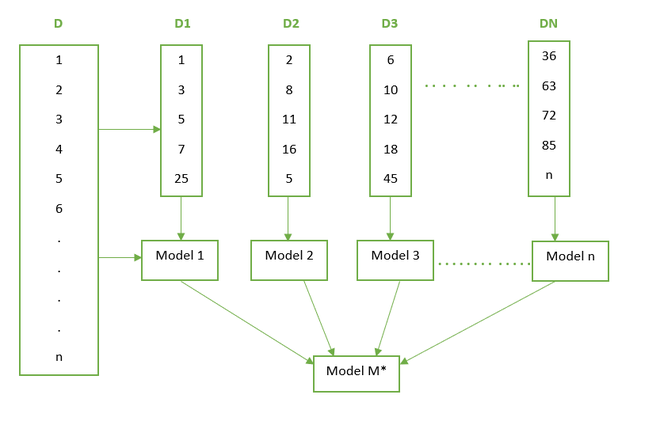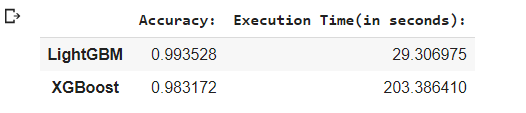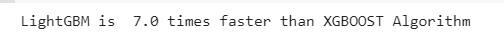Open in App
Not now

# LightGBM vs XGBOOST – Which algorithm is better

• Last Updated : 12 Feb, 2021

There are a lot of Data Enthusiasts who are taking part in a number of online competitive competitions in the domain of Machine Learning. Everyone has their own unique independent approach to determine the best model and predict the accurate output of the given problem statement. In Machine Learning, Feature Engineering is very much an integral part of the process and also consumes most of the time. On the other hand, Modeling becomes an important part where you cannot have much preprocessing or have certain constraints on the features. There are different ensemble methods that help in making strong robust models that can give very accurate predictions. But exactly what is this buzz of the word ‘Ensemble’? Let us understand what the term Ensemble means in detail.

Ensemble: Before moving into a technical definition straightaway let us take a simple real-life example and understand the same. Let us assume that you want to buy an electronic device – Mobile Phone. Your first approach would be searching on the Internet about different latest smartphones and comparing rates of different companies. You will also see the features in different models. After this first step, you will ask your friends about their opinion about the mobile phones which you have short-listed. In this fashion, you will take opinions and suggestions from a couple of people. Once you get a couple of positive reviews about a particular mobile phone you will go ahead and buy that mobile phone. This is the exact concept of the term ‘Ensemble’. Ensemble Methods is a machine learning technique in which several base models (weak learners) are combined in order to produce one powerful model. Let us now go further into detail with the Boosting Method.

Boosting: Boosting is one of the Sequential Ensemble techniques. This technique is usually applied to the data with high bias and low variance. Here we have a dataset ‘D’ with n records. We take a random sample of 5 records from the dataset. Here there is an equal probability of all the records to be selected. So, by random sampling, we have 1,3,5,7,25 records selected. We train a model (let us say decision tree) on this sample data. After that, we provide all the records of D dataset to this model to classify it. There would be some records that may be misclassified since Model 1 is a weak learner. The records misclassified are given more weight for the next sampling selection. So, these misclassified records will have a higher probability of selection than the other records in the dataset.  Here records 2,8, 1,16 were misclassified and record 5 also had a probability of selection so it got selected. Now again we train Model 2 and then provide the full data to the second model. This process is repeated for n models. These all models are weak learners. In the end, these models are aggregated and a final M* model is built which is a better performing model since the misclassification error has been minimized. The Below diagram represents the entire process neatly:So, having understood what is Boosting let us discuss the competition between the two popular boosting algorithms that is Light Gradient Boosting Machine and Extreme Gradient Boosting (xgboost). These algorithms yield the best results in a lot of competitions and hackathons hosted on multiple platforms. Let us now understand in-depth the Algorithms and have a comparative study on the same.

LGBM is a quick, distributed, and high-performance gradient lifting framework which is based upon a popular machine learning algorithm – Decision Tree. It can be used in classification, regression, and many more machine learning tasks. This algorithm grows leaf wise and chooses the maximum delta value to grow. LightGBM uses histogram-based algorithms. The advantages of this are as follows:

• Less Memory Usage
• Reduction in Communication Cost for parallel learning
• Reduction in Cost for calculating gain for each split in the decision tree.

So as LightGBM gets trained much faster but also it can lead to the case of overfitting sometimes. So, let us see what parameters can be tuned to get a better optimal model.

1. num_leaves: Since LightGBM grows leaf-wise this value must be less than 2^(max_depth) to avoid an overfitting scenario.
2. min_data_in_leaf: For large datasets, its value should be set in hundreds to thousands.
3. max_depth: A key parameter whose value should be set accordingly to avoid overfitting.

For Achieving Better Accuracy following parameters must be tuned:

1. More Training Data Added to the Model can increase accuracy. (can be also external unseen data)
2. num_leaves: Increasing its value will increase accuracy as the splitting is taking leaf-wise but overfitting also may occur.
3. max_bin: High value will have a major impact on accuracy but will eventually go to overfitting.

## XGBOOST Algorithm:

A very popular and in-demand algorithm often referred to as the winning algorithm for various competitions on different platforms. XGBOOST stands for Extreme Gradient Boosting. This algorithm is an improved version of the Gradient Boosting Algorithm. The base algorithm is Gradient Boosting Decision Tree Algorithm. Its powerful predictive power and easy to implement approach has made it float throughout many machine learning notebooks.  Some key points of the algorithm are as follows:

1. It does not build the full tree structure but builds it greedily.
2. As compared to LightGBM it splits level-wise rather than leaf-wise.
3. In Gradient Boosting, negative gradients are taken into account to optimize the loss function but here Taylor’s expansion is taken into account.
4. The regularization term penalizes from building complex tree models.

Some parameters which can be tuned to increase the performance are as follows:

General Parameters include the following:

1. booster: It has 2 options — gbtree and gblinear.
2. silent: If kept to 1 no running messages will be shown while the code is executing.
3. nthread: Mainly used for parallel processing. The number of cores is specified here.

Booster Parameters include the following:

1. eta: Makes model robust by shrinkage of weights at each step.
2. max_depth: Should be set accordingly to avoid overfitting.
3. max_leaf_nodes: If this parameter is defined then the model will ignore max_depth.
4. gamma: Specifies the minimum loss reduction which is required to make a split.
5. lambda: L2 regularization term on the weights.

Learning Task Parameters include the following:

1) objective: This will define the loss function which is to be used.

• binary: logistic –logistic regression for binary classification, returns predicted probability (not the class)
• multi: softmax –multiclass classification using the softmax objective, returns predicted class (not the probabilities)

2) seed: the default value set for this is zero. Can be used for parameter tuning.

So, we have gone through the basics of the algorithm and the parameters which need to be tuned for the respective algorithms. Now we will take a dataset and then compare both the algorithms on the basis of accuracy and execution time. Below is the Dataset Description which we are going to use:

Dataset Description:

The experiments have been carried out with a group of 30 volunteers within an age bracket of 19-48 years. Each person performed six activities (WALKING, WALKING_UPSTAIRS, WALKING_DOWNSTAIRS, SITTING, STANDING, LAYING) wearing a smartphone (Samsung Galaxy S II) on the waist. Using its embedded accelerometer and gyroscope, we captured 3-axial linear acceleration and 3-axial angular velocity at a constant rate of 50Hz. The experiments have been video-recorded to label the data manually. The obtained dataset has been randomly partitioned into two sets, where 70% of the volunteers were selected for generating the training data and 30% for the test data.

The sensor signals (accelerometer and gyroscope) were pre-processed by applying noise filters and then sampled in fixed-width sliding windows of 2.56 sec and 50% overlap (128 readings/window). The sensor acceleration signal, which has gravitational and body motion components, was separated using a Butterworth low-pass filter into body acceleration and gravity. The gravitational force is assumed to have only low-frequency components, therefore a filter with 0.3 Hz cutoff frequency was used. From each window, a vector of features was obtained by calculating variables from the time and frequency domain.

Python code implementation:

## Python3

 `# Importing the basic libraries``pip install pandas``import` `time``import` `pandas as pd``import` `numpy as np``import` `seaborn as sns``import` `matplotlib.pyplot as plt``%``matplotlib inline``import` `gc`` ` `gc.enable()`` ` `# Importing LGBM and XGBOOST``import` `lightgbm as lgb``import` `xgboost as xgb`` ` `# Reading the Data and Inspecting it``data``=``pd.read_csv(``'/content/drive/MyDrive/GeeksforGeeks_Datasets/smartphone_activity_dataset.csv'``)``data`

## Python3

 `# Checking for missing values across the 562 features of the dataset``data.isna().``sum``()``*``100``/``len``(data)`` ` `# Checking for Target Distribution``sns.countplot(data[``'activity'``])``plt.show()`

## Python3

 `# Saving the target variable(dependent variable) to target ``# variable and dropping it from the original "data" dataframe``target``=``data[``'activity'``]``data.drop(columns``=``{``'activity'``},inplace``=``True``)`` ` `# As the dataset contains all numerical features and also ``# the target classes are also encoded there is no more preprocessing``# needed at this stage. There are no missing values in the ``# dataset hence no missing data imputation is being needed. ``# Let us split the dataset and move to the modeling part. `` ` `# Splitting the Dataset into Training and Testing Dataset``from` `sklearn.model_selection ``import` `train_test_split``X_train,X_test,y_train,y_test``=``train_test_split(``  ``data,target,test_size``=``0.15``,random_state``=``100``)`` ` `# Applying the XGBOOST Model``# Setting Parameters required for XGBOOST Model ``# and Training the Model `` ` `# Starting to track the Time``start ``=` `time.time()   ``xg``=``xgb.XGBClassifier(max_depth``=``7``,learning_rate``=``0.05``,``                     ``silent``=``1``,eta``=``1``,objective``=``'multi:softprob'``,``                     ``num_round``=``50``,num_classes``=``6``) `` ` `# Setting the Parameters. More parameters can be set``# according to the requirement. ``# Here we have set the main parameters.`` ` `# Fitting the Model``xg.fit(X_train,y_train) `` ` `# Stopping the tracking of time  ``stop ``=` `time.time()    ``exec_time_xgb``=``stop``-``start`` ` `# Measuring the time taken for the model to build ``exec_time_xgb              `` ` `# Predicting the Output Class``ypred_xgb``=``xg.predict(X_test) ``ypred_xgb`` ` ` ` `# Getting the Accuracy Score for the XGBOOST Model``from` `sklearn.metrics ``import` `accuracy_score``accuracy_xgb ``=` `accuracy_score(y_test,ypred_xgb)`` ` `# Setting the Parameters and Training data for LightGBM Model``data_train ``=` `lgb.Dataset(X_train,label ``=` `y_train)``params``=` `{}`` ` `# Usually set between 0 to 1.``params[``'learning_rate'``]``=``0.5` ` ` `# GradientBoostingDecisionTree``params[``'boosting_type'``]``=``'gbdt'` ` ` `# Multi-class since the target class has 6 classes.``params[``'objective'``]``=``'multiclass'` ` ` `# Metric for multi-class``params[``'metric'``]``=``'multi_logloss'` `params[``'max_depth'``]``=``7``params[``'num_class'``]``=``7` ` ` `# This value is not inclusive of the end value.``# Hence we have 6 classes the value is set to 7.`` ` `# Training the LightGBM Model``num_round ``=``50``start ``=` `time.time()``lgbm ``=` `lgb.train(params,data_train,num_round)``stop ``=` `time.time()`` ` `#Execution time of the LightGBM Model``exec_time_lgbm ``=` `stop``-``start``exec_time_lgbm`` ` `# Predicting the output on the Test Dataset ``ypred_lgbm ``=` `lgbm.predict(X_test)``ypred_lgbm``y_pred_lgbm_class ``=` `[np.argmax(line) ``for` `line ``in` `ypred_lgbm]`

## Python3

 `# Accuracy Score for the LightGBM Model``from` `sklearn.metrics ``import` `accuracy_score``accuracy_lgbm``=``accuracy_score(y_test,y_pred_lgbm_class)`` ` `# Comparing the Accuracy and Execution Time for both the Algorithms``comparison ``=` `{``'Accuracy:'``:(accuracy_lgbm,accuracy_xgb),\``              ``'Execution Time(in seconds):'``:(exec_time_lgbm,exec_time_xgb)}``LGBM_XGB ``=` `pd.DataFrame(comparison)``LGBM_XGB .index ``=` `[``'LightGBM'``,``'XGBoost'``]``LGBM_XGB`` ` `# On comparison we notice that LightGBM is ``# faster and gives better accuracy.``comp_ratio``=``(``203.594708``/``29.443264``)``comp_ratio``print``(``"LightGBM is "``+``" "``+``str``(np.ceil(comp_ratio))``+``" "``+``\``      ``str``(``"times"``)``+``" "``+``"faster than XGBOOST Algorithm"``)`

Final Output:My Personal Notes arrow_drop_up